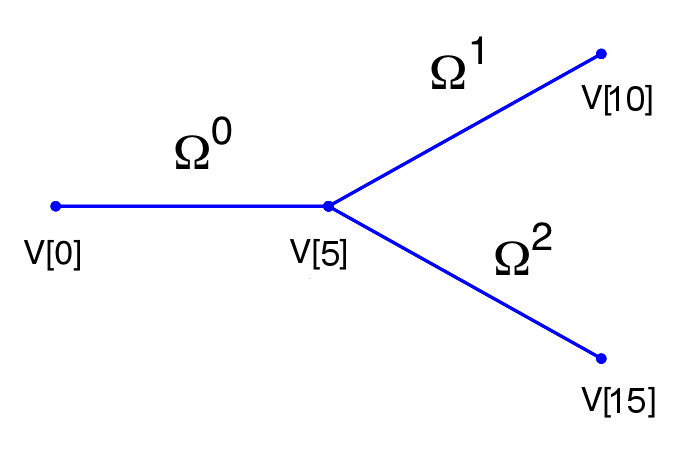### 12.3 Session file configuration

#### 12.3.1 Pulse Wave Sovler mesh connectivity

Typically 1D arterial networks are made up of a connection of different base units: segments, bifurcations and merging junctions. The input format in the PulseWaveSolver means these connections are handle naturally from the mesh topology; hence care must be taken when designing the 1D domain. The figure below outlines the structure of a bifurcation, which is a common reoccurring structure in the vasculature.Figure 12.1: Model of bifurcating artery. The bifurcation is made of three domains and 15 vertices. Vertex V is the inlet and vertices V and V the outlets.

To represent this topology in the xml file we specify the following vertices under the section `VERTEX` (the extents are: -100 ≥ x ≤ 100 and -100 ≥ y ≤ 100 )

1<VERTEX>
2  <V ID="0">-1.000e+02 0.000e+00 0.000e+00</V>
3  <V ID="1">-8.000e+01 0.000e+00 0.000e+00</V>
4  <V ID="2">-6.000e+01 0.000e+00 0.000e+00</V>
5  <V ID="3">-4.000e+01 0.000e+00 0.000e+00</V>
6  <V ID="4">-2.000e+01 0.000e+00 0.000e+00</V>
7  <V ID="5"> 0.000e+00 0.000e+00 0.000e+00</V>
8
9  <V ID="6"> 2.000e+01 2.000e+01 0.000e+00</V>
10  <V ID="7"> 4.000e+01 4.000e+01 0.000e+00</V>
11  <V ID="8"> 6.000e+01 6.000e+01 0.000e+00</V>
12  <V ID="9"> 8.000e+01 8.000e+01 0.000e+00</V>
13  <V ID="10"> 1.000e+02 1.000e+02 0.000e+00</V>
14
15  <V ID="11"> 2.000e+01 -2.000e+01 0.000e+00</V>
16  <V ID="12"> 4.000e+01 -4.000e+01 0.000e+00</V>
17  <V ID="13"> 6.000e+01 -6.000e+01 0.000e+00</V>
18  <V ID="14"> 8.000e+01 -8.000e+01 0.000e+00</V>
19  <V ID="15"> 1.000e+02 -1.000e+02 0.000e+00</V>
20</VERTEX>

The elements from these vertices are then constructed under the section `ELEMENT` by defining

1<ELEMENT>
2  <!-- Parent artery -->
3  <S ID="0">    0     1 </S>
4  <S ID="1">    1     2 </S>
5  <S ID="2">    2     3 </S>
6  <S ID="3">    3     4 </S>
7  <S ID="4">    4     5 </S>
8  <!-- Daughter artery 1 -->
9  <S ID="5">    5     6 </S>
10  <S ID="6">    6     7 </S>
11  <S ID="7">    7     8 </S>
12  <S ID="8">    8     9 </S>
13  <S ID="9">    9     10 </S>
14  <!-- Daughter artery 2 -->
15  <S ID="11">     5     11 </S>
16  <S ID="12">    11    12 </S>
17  <S ID="13">    12    13 </S>
18  <S ID="14">    13    14 </S>
19  <S ID="15">    14    15 </S>
20</ELEMENT>

The composites, which represent groups of elements and boundary regions are defined under the section `COMPOSITE` by

1<COMPOSITE>
2  <C ID="0"> S[0-4] </C>        <!-- Parent artery -->
3  <C ID="1"> V </C>          <!-- Inlet to domain -->
4
5  <C ID="3"> S[5-9] </C>        <!-- Daughter artery 1 -->
6  <C ID="4"> V </C>         <!-- Outlet of daughter artery 1 -->
7
8  <C ID="6"> S[11-15] </C>      <!-- Daughter artery 2 -->
9  <C ID="8"> V </C>         <!-- Outlet of daughter artery 2 -->
10</COMPOSITE>

Each of the segments can be then represented under the section `DOMAIN` by

1<DOMAIN>
2  <D ID="0"> C </D>  <!-- Parent artery -->
3  <D ID="1"> C </D>  <!-- Daughter artery 1 -->
4  <D ID="2"> C </D>  <!-- Daughter artery 2 -->
5</DOMAIN>

We will use the different domains later to define variable material properties and cross-sectional areas.

#### 12.3.2 Session Info

The PulseWaveSolver is sqpecified through the `EquationType` option in the session file. This can be set as follows:

• `Projection`: Only a discontinuous projection can be specified using the following option:
• `Discontinuous` for a discontinous Galerkin (DG) projection.
• `TimeIntegrationMethod`
• `UpwindTypePulse`:
• `UpwindPulse`

#### 12.3.3 Parameters

The following parameters can be specified in the `PARAMETERS` section of the session file.

• `TimeStep` is the time-step size;
• `FinTime` is the final physical time at which the simulation will stop;
• `NumSteps` is the equivalent of `FinTime` but instead of specifying the physical final time the number of time-steps is defined;
• `IO_CheckSteps` sets the number of steps between successive checkpoint files;
• `IO_InfoSteps` sets the number of steps between successive info stats are printed to screen;
• `rho` density of the fluid. Default value = 1.0;
• `nue` Poisson’s ratio. Default value = 0.5 ;
• `pest` external pressure. Default value = 0;
• `h0` wall thickness Default value = 1.0;

#### 12.3.4 Boundary conditions

In this section we can specify the boundary conditions for our problem. First we need to define the variables under the section `VARIABLES`.

1<VARIABLES>
2   <V ID="0"> A </V>
3   <V ID="1"> u </V>
4</VARIABLES>

The composites that we want to apply out boundary conditions then need to be defined in the `BOUNDARYREIONS`, for example if we had three composites (C, C and C) that correspond to three vertices of the computational mesh we would define:

1<BOUNDARYREGIONS>
2  <B ID="0"> C </B>
3  <B ID="1"> C </B>
4  <B ID="2"> C </B>
5 </BOUNDARYREGIONS>

Finally we can specify the boundary conditions on the regions specified under `BOUNDARYREGIONS`.

The Pulse Wave Solver comes with a number of boundary conditions that are unique to this solver. Boundary conditions must be provided for both the area and velocity at the inlets and outlets of the domain. Examples of the different boundary conditions will be provided in the following.

Inlet boundary condition:  Typically at the inlet of the domain a flow profile (`Q-inflow`) is specified through a `USERDEFINEDTYPE` boundary conditioning . An example inlet condition for the parent artery of the previously bifurcation example is

1<REGION REF="0">
2        <D VAR="A" USERDEFINEDTYPE="Q-inflow" VALUE="(7.112e-4)*(sin(7.854*t)
3-0.562)*(1/(1+exp(-400*(sin(7.854*t)-0.562))))" />
4        <D VAR="u" USERDEFINEDTYPE="Q-inflow" VALUE="1.0" />
5</REGION>

Terminal boundary conditions:  At the outlets of the domain there are four possible boundary conditions: reflection (`Terminal`), terminal resistance `R-terminal`, Two element windkessel (CR) `CR-terminal`, and three element windkessel (RCR) `RCR-terminal`. An example of the outflow boundary condition of the RCR terminal is given below

1<REGION REF="1">
2        <D VAR="A" USERDEFINEDTYPE="RCR-terminal" VALUE="RT" />
3        <D VAR="u" USERDEFINEDTYPE="RCR-terminal" VALUE="C" />
4</REGION>

Where `RT` is the total peripheral resistance used in the the `R-terminal`, `CR-terminal` and `RCR-terminal` models

#### 12.3.5 Functions

The following functions can be specified inside the `CONDITIONS` section of the session file:

• `MaterialProperties`: specifies the material properties for each domain.
• `A_0`: Initial area of each domain.
• `AdvectionVelocity`: specifies the advection velocity v.
• `InitialConditions`: specifies the initial condition for unsteady problems.
• `Forcing`: specifies the forcing function f

As an example to specify the material properties for each domain in the previous bifurcation example we would enter:

1<FUNCTION NAME="MaterialProperties">
2   <E VAR="beta" DOMAIN="0" VALUE="97" />
3   <E VAR="beta" DOMAIN="1" VALUE="87" />
4   <E VAR="beta" DOMAIN="2" VALUE="233" />
5</FUNCTION>

The values of `beta` are used in the pressure-area relationship (equation 12.2).